# Sort with SMALL & LARGE Functions in Excel & Google Sheets

This tutorial will demonstrate how to Sort by using Excel Functions, instead of the Sort Tool.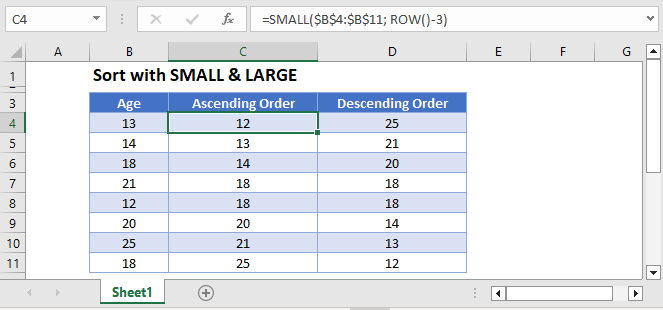## SMALL & LARGE Functions

We will use the SMALL (or LARGE) function along with the ROW Function to sort our data.

The SMALL Function returns the k-th smallest number from a set of data:

``=SMALL(B2:B8, 3)``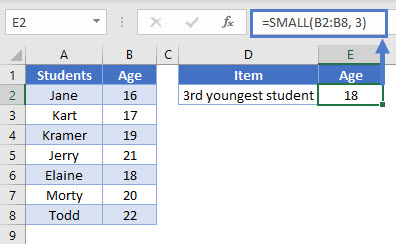While LARGE Function returns the k-th largest number:

``=LARGE(B2:B8, 3)``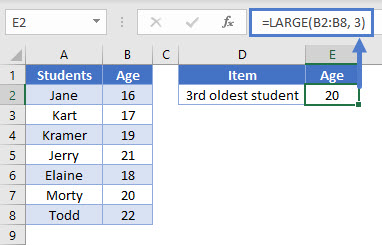We will use the SMALL Function to sort in ascending order and the LARGE Function to sort in descending order.

## ROW Function

We also need to use the ROW Function. The ROW Function returns the row number of a cell reference.

``= ROW(C8)``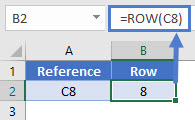It can also provide the row number of the cell where the formula was entered in.

``= ROW ()``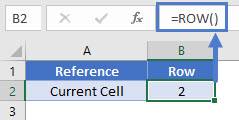## Sort Data In Ascending Order

We can sort data in ascending order using the SMALL Function and the ROW Function like this:

``=SMALL(\$A\$2:\$A\$9, ROW()-1)``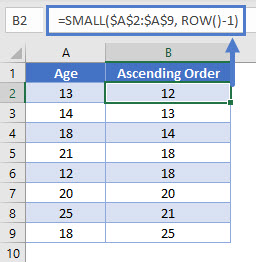### How does the formula work?

Remember this is our formula:

``=SMALL(A2:A9, ROW() - 1)``

It works by using the  to enter the correct k-value for each row. To sort the data in ascending order, the k-value should be 1 for the first cell and then increase as we go down the column.

In the first cell,

``````= SMALL(A2:A9, ROW(B2) - 1)
= SMALL (A2:A9, 2 - 1)
= SMALL (A2:A9, 1)``````

Similarly in the second cell,

``````= SMALL(A2:A9, ROW(B3) - 1)
= SMALL (A2:A9, 3 - 1)
= SMALL (A2:A9, 2)``````

In this way, the k-value increases as we go down the column, outputting the numbers from smallest to largest.

## Sort Data In Descending Order

Similarly, we can sort data in descending order using the LARGE Function and the ROW Function. It works exactly like the formula above.

``=LARGE(\$A\$2:\$A\$9, ROW()-1)``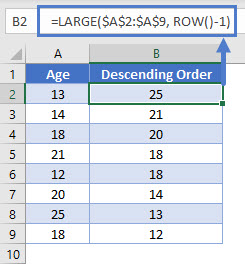Note: The above formula uses absolute references (the \$ signs) to lock cell references when copying formulas. If you aren’t familiar with this, please read our Excel References Guide.

## Google Sheets –Sort with SMALL & LARGE Functions

All of the above examples work exactly the same in Google Sheets as in Excel.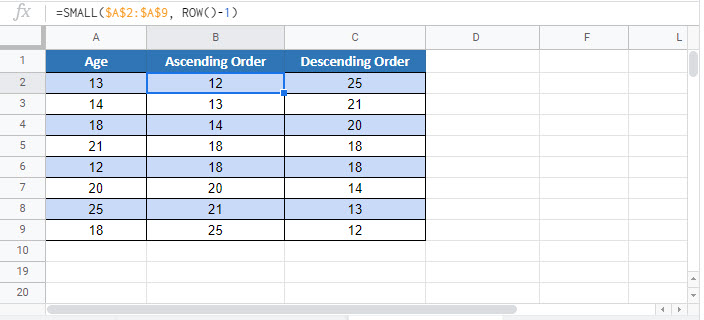### Excel Practice Worksheet

Practice Excel functions and formulas with our 100% free practice worksheets!